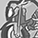# QlikView App Dev

Discussion Board for collaboration related to QlikView App Development.

Announcements
Action-Packed Learning Awaits! QlikWorld 2023. April 17 - 20 in Las Vegas: REGISTER NOW
cancel
Showing results for
Did you mean:Specialist

## Dimensionality for custom subtotal in pivot table

Hi All,

I am trying to modify a subtotal using Dimensioanlity:

In case a dimension has the value 3, to sum items from dimension 2 and 3, which are flagged in the script.

=if(Dimensionality() =2 and Balance_TotalCode =3, sum({<Balance_Flag_TOTALCOMMANDEQU={'1'}>}Balance_Amount),sum(Balance_Amount))

In the data:It should sum the items in orange (Balance_TotalCode =2) and green (Balance_TotalCode =3)

But It only sums the items in green.

The row in yellow represents the desired location with the wrong result.Will appreciate assistance!

Thanks!

1 Solution

Accepted SolutionsMVP

Try with Total qualifier

=If(Dimensionality() =2 and Balance_TotalCode =3, Sum(TOTAL {<Balance_Flag_TOTALCOMMANDEQU = {'1'}>} Balance_Amount), Sum(Balance_Amount))

3 RepliesMVP

Try with Total qualifier

=If(Dimensionality() =2 and Balance_TotalCode =3, Sum(TOTAL {<Balance_Flag_TOTALCOMMANDEQU = {'1'}>} Balance_Amount), Sum(Balance_Amount))Specialist
Author

Kudos Sunny!

As usual, your help is tremendous!Creator II

Hi Experts,

I am new to learn this topic,

can you please share the sample QVW file.Community Browser